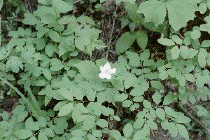# Biology

#### Biology Lab: Properties of WaterLab Exercise

# Biology Lab: Properties of Water

There is no equivalent lab in the Illustrated Guide to Home Biology Experiments. If you are interested in further biology-related water properties investigation, you might take a look at Mr. Dougherty's Biology Course Water Lab.

## Goal: To observe and identify various properties of water.

You may do either A or B or both, depending on the amount of time that you have available. If you do both, report them together as a single lab.

### Materials for A: Boiling Water

• Thermometer capable of reading from 20F to 220F or from -10 to +110C
• 2-3 quart sauce or other pot
• Supply of water
• Heat source, such as a stove
• Hot pads or heat proof mittens, tongs and clamps suitable for grabbing hot items

### Materials for B: Surface Tension

• Bowl for water
• Supply of water
• Needle
• Scale to measure mass

### Procedure

1. The temperature of boiling water
1. You are going to observe the temperature of water as you heat it to and past the boiling point. Write a hypothesis of how you expect the temperature to change.
2. Fill the pot 2/3 full of water (the exact amount is immaterial, but you may want to record it).
3. Record the starting temperature of the water using your thermometer. Be sure to use heat-proof mittens or a clamp to handle the thermometer: hot glass looks just like cold glass.
4. Put the water over your heat source.
5. You will record the time of observation and temperature of the water at that time. Design a table on which to capture and present this information. If you have one available, use a spreadsheet program.
6. Make an observation of the temperature every minute, noting when the water starts to boil.
7. After the water begins to boil, note the temperature of the water every minute for three more minutes.
1. On a piece of graph paper, draw a vertical line on the left side. Make a scale that goes from 10 degrees below your starting temperature to 10 degrees above the highest temperature you recorded.
2. At the bottom of the page, draw a line and make a scale that goes from zero to two more than the highest number of minutes for which you recorded time.
3. Plot the temperature against the time at which you measured it.
4. Draw the best curve you can manage through your plotted points.
5. Describe your curve in words.
9. Determine the average rate of change in the temperature of the water before and after boiling (in degrees per minute)?
1. Add three columns (or rows, depending on how you set up your table) to your table.
2. In one, calculate the change in time between each measurement and the next.
3. In the second, calculate the change in temperature.
4. In the third, calculate the rate of change by dividing the change in temperature by the change in time.
5. Add up the individual rates and divide them by the number of rates to determine the average.
10. Determine the amount of error in your measurement.
1. Add another column to your table, and determine the difference between the rate for the individual measurements and the average. This is the deviation of the measurement.
2. Add up the deviations and determine the number of them; this is the average deviation.
3. If you know how do do this on your or spreadsheet, determine the standard deviation (check the instructions for your own calculator; methods vary depending on how your calculator or spreadsheet is programmed).
11. Repeat the experiment two more times, using the same amount of water.
12. Predict what will happen if you use half the water; test your prediction.
13. What happens to the temperature when the water starts to boil? Explain what is happening to the heat and the water.
2. Surface tension
1. Place an inch of water in a small bowl, such as a cereal bowl.
2. Very carefully float a sewing needle on the surface of the water (it may take you several tries to get this to work). Do not use a pin with a head--the head may pierce the surface and pull the pin down into the water. Do not use a toothpick or anything which would normally float if submerged completely first.
3. Carefully describe the appearance of the surface of the water near and under the needle. Why does the heavy needle float?
4. Add a drop of detergent to the water. What happens?
5. Devise an experiment to determine how much weight the surface tension of clean water can support. Run your experiment, and present your data, error determinations, and conclusions.